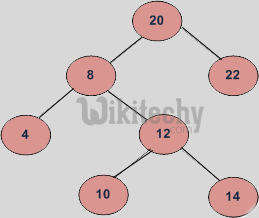# Inorder Successor in Binary Search Tree

Python Program - In order Successor in Binary Search Tree - Binary Search Tree - In order Successor is NULL for the last node in In order traversal.

Inorder Successor in Binary Search Tree:

In Binary Tree, Inorder successor of a node is the next node in Inorder traversal of the Binary Tree. Inorder Successor is NULL for the last node in Inorder traversal.

In Binary Search Tree, Inorder Successor of an input node can also be defined as the node with the smallest key greater than the key of input node. So, it is sometimes important to find next node in sorted order.In the above diagram, inorder successor of 8 is 10, inorder successor of 10 is 12 and inorder successor of 14 is 20.

### Method 1 (Uses Parent Pointer)

In this method, we assume that every node has parent pointer.

The Algorithm is divided into two cases on the basis of right subtree of the input node being empty or not.

Input: node, root // node is the node whose Inorder successor is needed.
output: succ // succ is Inorder successor of node.

1) If right subtree of node is not NULL, then succ lies in right subtree. Do following.
Go to right subtree and return the node with minimum key value in right subtree.
2) If right subtree of node is NULL, then succ is one of the ancestors. Do following.
Travel up using the parent pointer until you see a node which is left child of it’s parent. The parent of such a node is the succ.

### Implementation of Python Program:

Note that the function to find InOrder Successor is highlighted (with gray background) in below code.

Python Programming
``````# Python program to find the inroder successor in a BST

# A binary tree node
class Node:

# Constructor to create a new node
def __init__(self, key):
self.data = key
self.left = None
self.right = None

def inOrderSuccessor(root, n):

# Step 1 of the above algorithm
if n.right is not None:
return minValue(n.right)

# Step 2 of the above algorithm
p = n.parent
while( p is not None):
if n != p.right :
break
n = p
p = p.parent
return p

# Given a non-empty binary search tree, return the
# minimum data value found in that tree. Note that the
# entire tree doesn't need to be searched
def minValue(node):
current = node

# loop down to find the leftmost leaf
while(current is not None):
if current.left is None:
break
current = current.data

return current

# Given a binary search tree and a number, inserts a
# new node with the given number in the correct place
# in the tree. Returns the new root pointer which the
# caller should then use( the standard trick to avoid
# using reference parameters)
def insert( node, data):

# 1) If tree is empty then return a new singly node
if node is None:
return Node(data)
else:

# 2) Otherwise, recur down the tree
if data <= node.data:
temp = insert(node.left, data)
node.left = temp
temp.parent = node
else:
temp = insert(node.right, data)
node.right = temp
temp.parent = node

# return  the unchanged node pointer
return node

# Driver progam to test above function

root  = None

# Creating the tree given in the above diagram
root = insert(root, 20)
root = insert(root, 8);
root = insert(root, 22);
root = insert(root, 4);
root = insert(root, 12);
root = insert(root, 10);
root = insert(root, 14);
temp = root.left.right.right

succ = inOrderSuccessor( root, temp)
if succ is not None:
print "\nInorder Successor of %d is %d " \
%(temp.data , succ.data)
else:
print "\nInorder Successor doesn't exist"

# This code is contributed by Nikhil Kumar Singh(nickzuck_007)``````

### Output:

Inorder Successor of 14 is 20

Time Complexity: O(h) where h is height of tree.

### Method 2 (Search from root)

Parent pointer is NOT needed in this algorithm. The Algorithm is divided into two cases on the basis of right subtree of the input node being empty or not.

Input: node, root // node is the node whose Inorder successor is needed.
output: succ // succ is Inorder successor of node.

Step 1: If right subtree of node is not NULL, then successor lies in right subtree. Do following.
Go to right subtree and return the node with minimum key value in right subtree.
Step 2: If right subtree of node is NULL, then start from root and us search like technique. Do following.
Travel down the tree, if a node’s data is greater than root’s data then go right side, otherwise go to left side.

#### About the author#### Venkatesan Prabu

Wikitechy Founder, Author, International Speaker, and Job Consultant. My role as the CEO of Wikitechy, I help businesses build their next generation digital platforms and help with their product innovation and growth strategy. I'm a frequent speaker at tech conferences and events.